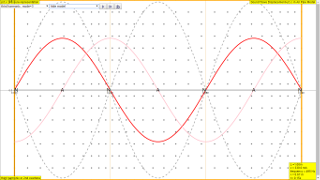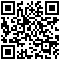## Topics

Transverse and longitudinal waves
Determination of frequency and wavelength
Stationary waves

## Description

In this Open/Closed Pipe wave model, lets consider a narrow pipe along the OX axis. The simulation will display the first 5 normal modes, which are
From the drop-down menu, the mode n = 1, ...,5, may be selected.
Units are arbitrary set to be in SI unit for calculation purposes

The modeling equation is governed by u(t,x) where

• u(t,x) = A sin(n π x) cos(ω t + δ) when both ends are closed.
• u(t,x) = A sin((n-1/2) π x) cos(ω t + δ) when the left end is closed and the right end open.
• u(t,x) = A cos((n-1/2) π x) cos(ω t + δ) when the left end is open and the right end closed.
• u(t,x) = A cos(n π x) cos(ω t + δ) when both ends are open.

Play with the Model. Test what you've learned by exploring the amplitudes, wavelengths, periods, frequencies and  wave velocities.

## Sample Learning Goals

(a) show an understanding and use the terms displacement, amplitude, phase difference, period,
frequency, wavelength and speed
(b) deduce, from the definitions of speed, frequency and wavelength, the equation v = fλ
(c) recall and use the equation v = fλ
(f) analyse and interpret graphical representations of transverse and longitudinal waves
(i) determine the wavelength of sound using stationary waves.
(a) explain and use the principle of superposition in simple applications
(b) show an understanding of experiments which demonstrate stationary waves using microwaves, stretched strings and air columns
(c) explain the formation of a stationary wave using a graphical method, and identify nodes and antinodes

## Activities

1. Compute the position of the nodes(always zero displacement) for mode number n=1,2,3,4,5 cases for an open-open end system of the air in the pipe.
2. Use the simulation to check your calculation.
3. select the correct modeling equation for fixed end string n=1,2,3,4,5 respectively.
4. how can you suggest is the parameter that describe these series of stationary waves.

Introduction

# Standing waves in a pipe

Let us consider a narrow pipe along the OX axis. Each end may be open or closed. The simulation will display the first 5 normal modes, which are
1. u(t,x) = A sin(n π x) cos(ω t + δ) when both ends are closed.
2. u(t,x) = A sin((n-1/2) π x) cos(ω t + δ) when the left end is closed and the right end open.
3. u(t,x) = A cos((n-1/2) π x) cos(ω t + δ) when the left end is open and the right end closed.
4. u(t,x) = A cos(n π x) cos(ω t + δ) when both ends are open.
• Units are arbitrary.
• Below you may choose the mode n = 1, ...,5, as well as the animation step Δt.
• The upper animation shows the displacement field u(t,x) and the pressure p(t,x) as functions of x at each time t.
• In the lower animation you may see the evolution of the position x + u(t,x) of several points and a contour plot of p(t,x) (lighter/darker blue means higher/lower pressure).
• Optionally one can see the nodes where the displacement wave vanishes at all times.
• Scale has been arbitrarily enhanced to make things visible; but keep in mind that we are considering very small displacements and pressure changes in a narrow pipe.
• Put the mouse point over an element to get the corresponding tooltip.
Activities

## Activities

• Compute the position of the nodes for mode number n in the four considered cases.
• Use the simulation to check your calculation.
• Where are the pressure nodes in the different cases?
• Which is the relationship between the displacement and pressure waves? How does it appears in the animation?
Author This is an English translation of the Basque original for a course on mechanics, oscillations and waves.
It requires Java 1.5 or newer and was created by Juan M. Aguirregabiria with Easy Java Simulations (Ejs) by Francisco Esquembre. I thank Wolfgang Christian and Francisco Esquembre for their help.

### Translations

Code Language Translator Run### Software Requirements

SoftwareRequirements

 Android iOS Windows MacOS with best with Chrome Chrome Chrome Chrome support full-screen? Yes. Chrome/Opera No. Firefox/ Samsung Internet Not yet Yes Yes cannot work on some mobile browser that don't understand JavaScript such as..... cannot work on Internet Explorer 9 and below

### CreditsJuan M. Aguirregabiria (http://tp.lc.ehu.es/jma.html); lookang; tina

### Challenging Questions

Q1: Where does the greatest pressure occur?

A1: During compression, the molecules of the medium are forced together, resulting in the increased pressure and density. During expansion the molecules are forced apart, resulting in the decreased pressure and density. This coincide with the points of permanent zero amplitudes which are also called nodes. Refer to the simulations as N, nodes.

### Other Hands-on Kit

1. https://itunes.apple.com/us/app/spectrumview/id472662922?mt=8 contributed by Dave
2. https://play.google.com/store/apps/details?id=processing.test.soundanalyzer contributed by Leong Tze Kwang and worksheet Sound Wave Experiment.docx

### end faq

Rating 5.00 (1 Vote)
Parent Category: 04 Waves
Category: 02 General Waves
Hits: 6931